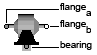To Space Phasor - MapleSim Help

To Space Phasor

Convert three-phase to space phasorDescription The To Space Phasor component converts a three-phase vector to a space phasor and zero (average) signal.Equations $y=Mu$ $M=\left(\begin{array}{ccc}1& -\frac{1}{2}& -\frac{1}{2}\\ 0& \frac{\sqrt{3}}{2}& -\frac{\sqrt{3}}{2}\end{array}\right)$ $\mathrm{zero}=\frac{1}{3}\left({u}_{1}+{u}_{2}+{u}_{3}\right)$Connections

 Name Description Modelica ID $u$ Real 3-vector input; 3-phase input u $y$ Real output 2-vector; space phasor y $\mathrm{zero}$ Real output; average zeroModelica Standard Library The component described in this topic is from the Modelica Standard Library. To view the original documentation, which includes author and copyright information, click here.